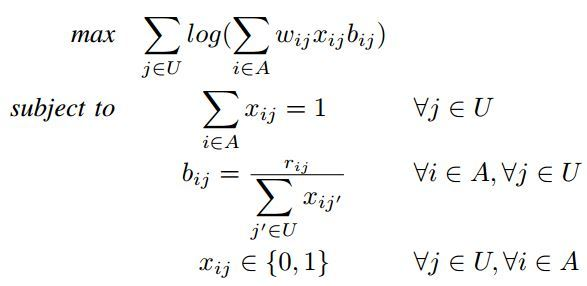# Solving multi variable logarithmic objective function

I am trying to solve the following problem using CVX. Indeed, someone has claimed that it is solved using CVX already and I would like to redo what has been done before:maximize \sum\sum

when I attempt the following code, I always get “Invalid quadratic form(s): not a square.” error and I am pretty stuck using CVX to deal with this problem.

r = [54 54 54;
54 54 54;
54 54 54;
54 54 54;
54 54 54];
[m,n] = size®; % m X Users & n X APs

cvx_begin
variable x(m,n)
variable b(m,n)
maximize sum(sum(x(1:m,1:n).*b(1:m,1:n) ))
subject to
sum(x,2) == 1
b == r ./ sum(x,1)
0 <= x <= 1
cvx_end

Regarding to the author, x can be considered as a real variable for relaxation and after solving the problem by using rounding technique we can solve the original problem. I really appreciate your feedback. thanks.

I have changed the format of the program as follows and I used geometric mode, but still I get error to compile the objective function! any feedback? (P.S.: I ignored log function at the moment)

Error using cvxprob/newobj (line 57)
Disciplined convex programming error:
Cannot maximize a(n) log-convex expression.

Error in maximize (line 21)
newobj( prob, ‘maximize’, x );

## Error in test (line 41) maximize x11b11 + x12b12 + x21b21 + x22b22;

cvx_begin gp
variables x11 x12 x21 x22
variables b11 b12 b21 b22
maximize x11*b11 + x12*b12 + x21*b21 + x22*b22;

subject to
0 <= x11 <= 1;
0 <= x12 <= 1;
0 <= x21 <= 1;
0 <= x22 <= 1;
x11 + x21 == 1;
x12 + x22 == 1;
b11*x11 + b11*x12 == 54;
b12*x11 + b12*x12 == 54;
b21*x21 + b21*x22 == 54;
b22*x21 + b22*x22 == 54;


cvx_end

guys! I expect to see something from you after a few days.

I’ll be happy to refund your purchase price.

this is not the professional way to answer a question. I expected at least to get some guides on similar problems.

Oh, I agree, it’s not professional. I wasn’t trying to be. I would suggest you recognize this forum is provided and paid for as a public service, and run on a volunteer basis. If we don’t have an answer for you, that’s just the way it is.

I see, so let’s be it and forget this post.